# DA_Converter

Simple digital to analog converter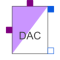# Information

This information is part of the Modelica Standard Library maintained by the Modelica Association.

Simple digital to analog converter with a variable input signal width of N bits. The input signal is an N-vector of type Logic (9-valued logic according to IEEE 1164 STD_ULOGIC). The output voltage of value `y` is generated by an ideal voltage source. The output can only change if the trigger signal `trig` of type Logic changes to '1' (forced or weak). In this case, the output voltage is calculated in the following way:

```       N
y = SUM ( x[i]*2^(i-1) )*Vref/(2^N-1),
i=1
```

where x[i], i=1,...,N is 1 or 0. and Vref is the reference value. Therefore, the first bit in the input vector x is the least significant one (LSB) and x[N] is the most significant bit (MSB).

This is an abstract model of a DAC. Hence, it can not cover the dynamic behaviour of the converter. Therefore the output will change instantaneously when the trigger signal rises.

# Parameters (2)

N Value: Type: Integer Description: Resolution - input signal width Value: Type: Voltage (V) Description: Reference voltage

# Connectors (4)

trig x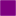Type: DigitalInput Description: Trigger inputType: DigitalInput[N] Description: Digital input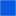Type: PositivePin Description: Positive electrical pin (output)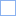Type: NegativePin Description: Negative electrical pin (output)

# Used in Examples (1)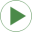AD_DA_conversion Modelica.Electrical.Analog.Examples Conversion circuit